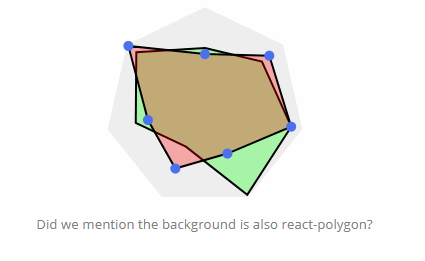/ Animation

# A react add-on for drawing polygons for any number of sides## react-polygon

A react add-on for drawing polygons for any number of sides, as well as animation.

# Usage

``````npm install react-polygon --save
``````
``````import Polygon from 'react-polygon'

<Polygon />
``````

``````npm install
npm run webpack
``````

# Props

name : propType = defaultValue

## Basic

• n : number = 3
• size : number = 50
• fill : string = "#ad893e"
• ratios : [number] = [1, 1, 1, 1, 1]

## Animation

• isAnimating : bool = true
• duration : number = 1000

## renderPoint

You can render extra elements on each point by passing a function to the props `renderPoint`. Here is an example for rendering a point on each vertice:

``````import React, { Component } from 'react'
import Polygon from 'react-polygon'

class MyPolygon extends Component {
myRenderPoint (point) {
return (
<circle cx={point} cy={point} r={5} />
)
}

render () {
return (
<Polygon renderPoint={this.myRenderPoint} />
)
}
}
``````

## GitHub#### React.js Examples

A nice collection of often useful examples done in React.js.

Latest
Tag